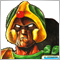# Draw rectangle around range of bars by hours303

Hello

This ought to be easy but I'm finding it fiendish.

I want to draw a rectangle around my non-trading hours.

I can do this ok in a custom indicator, by scanning the Bars-IndicatorCounted() for the start hour of my trading day, and when I get that, draw a rectangle from the endOfTradingDay bar to the startOfTradingBar-1, using the lowest low and highest high of the outOfHours bar range to draw the shape.

The trouble is at the right-hand, nascent end of the chart.  If my window is 1600-0800, if the time is e.g. 2300, the indicator won't have drawn the rectangle for the most recent time yet, as a bar with TimeHour()=8 won't have arrived yet.

Realising this, it meant that I need to create a rectangle at endOfTradingDay, and then ObjectModify point 1 of the rectangle every bar, so that if my outOfHours rectangles are normally 16 bars wide (historically), the most recent rectangle will 'grow' from 1600 while the time is overnight (our e.g. rectangle would be only 7 bars wide (23-16))

But to write the code for this eventuality meant I really needed to write one algorithm to cover both 'historical' rectangles and the nascent (right-most) narrower rectangle of the chart because the 'mascent' rectangle will eventually become 'historical'.  And with the notion of part of my rectangle being 1600->2400 and part being at the 0000->0800, and the start being after the beginning, I got confused and stuck.

I wondered if anyone had done something like this before and could share the function with me just to get me going again please?27515

strontiumDog:

The trouble is at the right-hand, nascent end of the chart.  If my window is 1600-0800, if the time is e.g. 2300, the indicator won't have drawn the rectangle for the most recent time yet, as a bar with TimeHour()=8 won't have arrived yet.

Realising this, it meant that I need to create a rectangle at endOfTradingDay, and then ObjectModify point 1 of the rectangle every bar,

You've answered your own question. If the current bar is in the window, find the window start, the low and high, create or modify the rectangle.
```#define HR2400 (PERIOD_D1 * 60)  // 86400 = 24 * 3600
#define INDEX   uint
#define SECONDS uint
SECONDS     time(datetime when=0){        if(when == 0) when = TimeCurrent();
return SECONDS(when % HR2400);
}
datetime    date(datetime when=0){        if(when == 0) when = TimeCurrent();
return datetime(when - time(when) );
}
/*
datetime    tomorrow( datetime when=0){   if(when == 0) when = TimeCurrent();
return date(when) + HR2400;
}
*/
INDEX       iYesterday(datetime when=0){  if(when == 0) when = TimeCurrent();
return iBarShift(_Symbol, PERIOD_CURRENT, date(when) - 1);
}
datetime    yesterday(datetime when=0){
return Time[iYesterday(when)];
}
bool        in_range(SECONDS beg, SECONDS end,
datetime when=0){ if(when == 0) when = TimeCurrent();
SECONDS  tod   = time(when);
return beg < end ? beg <= tod && tod < end : !in_range(end, beg, when);
}
//////////////////////////////////////////////////////////////////////////////
#define HRBEG 57600 // 1600
#define HREND 28000 // 0800
for(INDEX iBar=...){
if(in_range(HRBEG, HREND, Time[iBar]) ){
datetime bod   = date(Time[i]);     SECONDS  tod = time(Time[iBar]);
if (tod < HRBEG)  bod = yesterday(bod);
INDEX    iBeg  = iBarShift(_Symbol, PERIOD_CURRENT, bod + HRBEG);
int      n     = iBeg - iBar + 1;
INDEX    iLo   =  iLowest(_Symbol, PERIOD_CURRENT, MODE_LOW,  n, iBar);
INDEX    iHi   = iHighest(_Symbol, PERIOD_CURRENT, MODE_High, n, iBar);
draw_rectangle(PREFIX+Time[iBeg], Time[iBeg],  Low[iLo],
Time[iBar], High[iHi]);
}  // in_range
}  // for
```303

whroeder1:
You've answered your own question. If the current bar is in the window, find the window start, the low and high, create or modify the rectangle.
thanks27515

 Wrong except on the D1 ```INDEX       iYesterday(datetime when=0){  if(when == 0) when = TimeCurrent();    return iBarShift(_Symbol, PERIOD_CURRENT, date(when) - 1); }``` Correction ```INDEX       iYesterday(datetime when=0){  if(when == 0) when = TimeCurrent();    datetime today = date(when);    return iBarShift(_Symbol, PERIOD_CURRENT, date(today - 1)); }```1719

hello how do i draw a rectangle on the last 8 bars including a pinbar as first bar thank you. MT427515

You know (or should know) how. Get the index of the “pinbar.” Get the index of “the last 8 bars.” (Whatever that means.) Get the time and price of those two indexes. Draw the rectangle.

You haven't stated a problem, you stated a want. Show us your attempt (using the CODE button) and state the nature of your problem.
No free help 2017.04.21